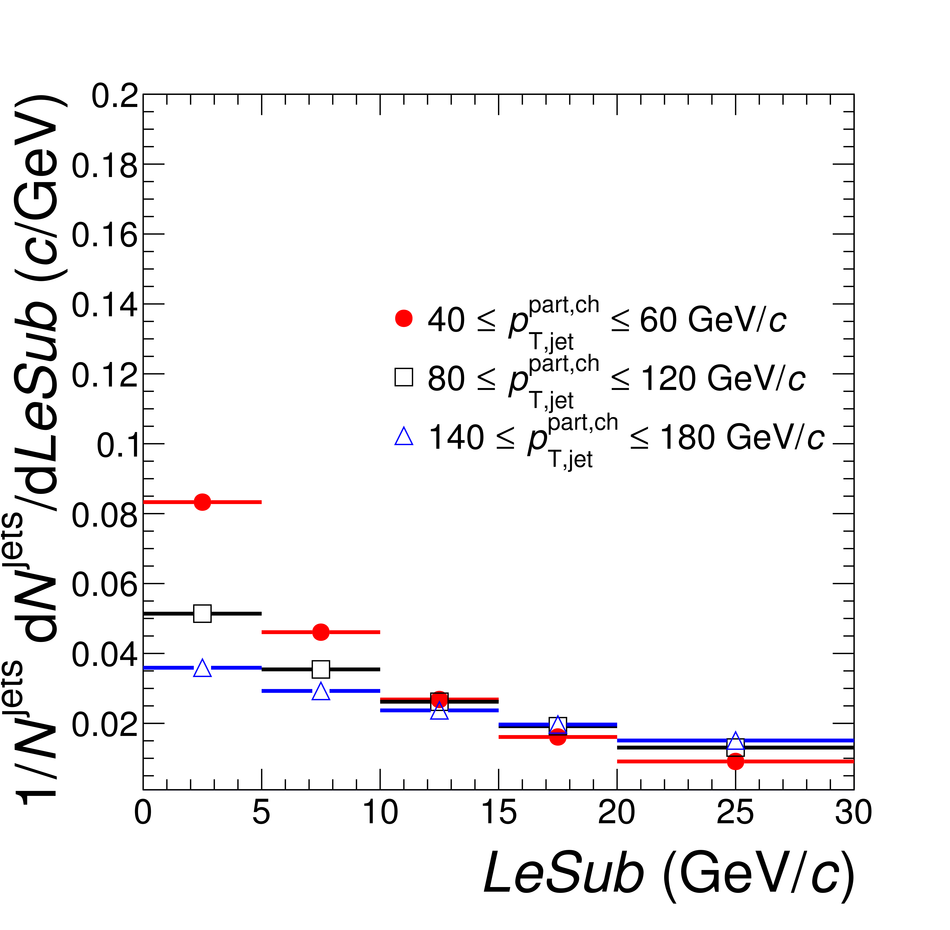# Figure 2

 $g$, $p_{\rm T}D$, and $LeSub$ as obtained from PYTHIA Perugia 2011 simulations of pp collisions at $\sqrt{s}=2.76$\,TeV for three different transverse momentum intervals.